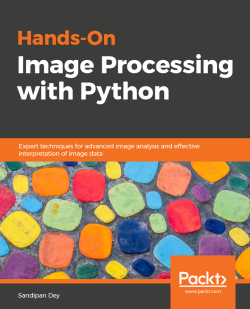# Hands-On Image Processing with Python3.5 (2 reviews total)
By Sandipan Dey
• \$37.99 eBook
• \$46.99 Print + eBook
• \$12.99 eBook + Subscription
What do you get with a Packt Subscription?

• Constantly updated with 100+ new titles each month
• Breadth and depth in over 1,000+ technologies

Image processing plays an important role in our daily lives with various applications such as in social media (face detection), medical imaging (X-ray, CT-scan), security (fingerprint recognition) to robotics & space. This book will touch the core of image processing, from concepts to code using Python.

The book will start from the classical image processing techniques and explore the evolution of image processing algorithms up to the recent advances in image processing or computer vision with deep learning. We will learn how to use image processing libraries such as PIL, scikit-mage, and scipy ndimage in Python. This book will enable us to write code snippets in Python 3 and quickly implement complex image processing algorithms such as image enhancement, filtering, segmentation, object detection, and classification. We will be able to use machine learning models using the scikit-learn library and later explore deep CNN, such as VGG-19 with Keras, and we will also use an end-to-end deep learning model called YOLO for object detection. We will also cover a few advanced problems, such as image inpainting, gradient blending, variational denoising, seam carving, quilting, and morphing.

By the end of this book, we will have learned to implement various algorithms for efficient image processing.

Publication date:
November 2018
Publisher
Packt
Pages
492
ISBN
9781789343731

## Chapter 1. Getting Started with Image Processing

As the name suggests, image processing can simply be defined as the processing (analyzing and manipulating) of images withalgorithms in a computer (through code). It has a few different aspects, such as storage, representation, information extraction, manipulation, enhancement, restoration, and interpretation of images. In this chapter, we are going to give a basic introduction to all of these different aspects of image processing, along with an introduction to hands-on image processing with Python libraries. We are going to use Python 3 for all of the code samples in this book.

We will start by defining what image processing is and what the applications of image processing are. Then we will learn about the basic image processing pipeline—in other words, what are the steps to process an image on a computer in general. Then, we will learn about different Python libraries available for image processing and how to install them in Python 3. Next, we will learn how to write Python codes to read and write (store) images on a computer using different libraries. After that, we will learn the data structures that are to be used to represent an image in Python and how to display an image. We will also learn different image types and different image file formats, and, finally, how to do basic image manipulations in Python.

By the end of this chapter, we should be able to conceptualize image processing, different steps, and different applications. We should be able to import and call functions from different image processing libraries in Python. We should be able to understand the data structures used to store different types of images in Python, read/write image files using different Python libraries, and write Python code to do basic image manipulations. The topics to be covered in this chapter are as follows:

• What image processing is and some image processing applications
• The image processing pipeline
• Setting up different image processing libraries in Python
• Image I/O and display with Python
• Image types, file formats, and basic image manipulations

## What is image processing and some applications

Let's start by defining what is an image, how it is stored on a computer, and how we are going to process it with Python.

### What is an image and how it is stored on a computer

Conceptually, an image in its simplest form (single-channel; for example, binary or mono-chrome, grayscale or black and white images) is a two-dimensional function f(x,y) that maps a coordinate-pair to an integer/real value, which is related to the intensity/color of the point. Each point is called a pixel or pel (picture element). An image can have multiple channels too (for example, colored RGB images, where a color can be represented using three channels—red, green, and blue). For a colored RGB image, each pixel at the (x,y) coordinate can be represented by a three-tuple (rx,y, gx,y, bx,y).

In order to be able to process it on a computer, an image f(x,y) needs to be digitalized both spatially and in amplitude. Digitization of the spatial coordinates (x,y) is called image sampling. Amplitude digitization is called gray-level quantization. In a computer, a pixel value corresponding to a channel is generally represented as an integer value between (0-255) or a floating-point value between (0-1). An image is stored as a file, and there can be many different types (formats) of files. Each file generally has some metadata and some data that can be extracted as multi-dimensional arrays (for example, 2-D arrays for binary or gray-level images and 3D arrays for RGB and YUV colored images). The following figure shows how the image data is stored as matrices for different types of image. As shown, for a grayscale image, a matrix (2-D array) of width x height suffices to store the image, whereas an RGB image requires a 3-D array of a dimension of width x height x 3: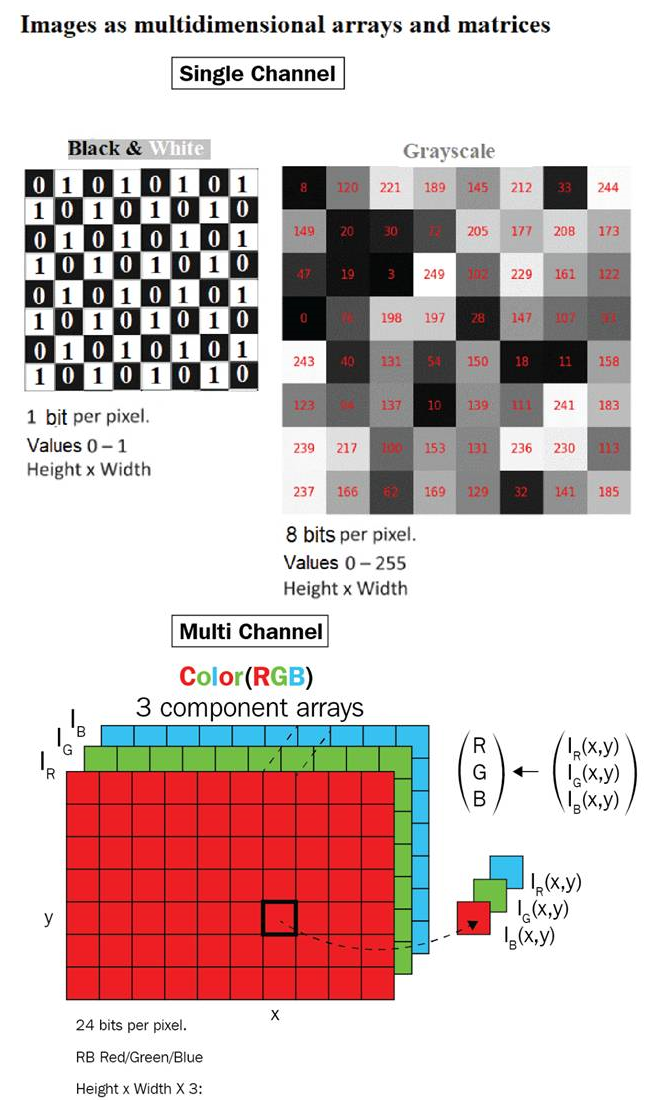The next figure shows example binary, grayscale, and RGB images: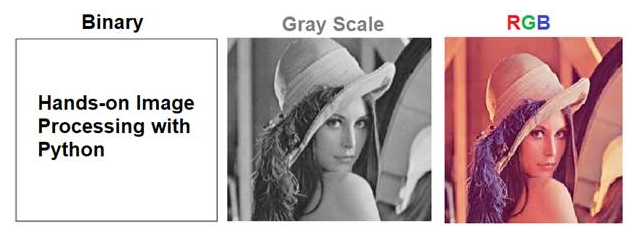In this book, we shall focus on processing image data and will use Python libraries to extract the data from images for us, as well as run different algorithms for different image processing tasks on the image data. Sample images are taken from the internet, from the Berkeley Segmentation Dataset and Benchmark (https://www2.eecs.berkeley.edu/Research/Projects/CS/vision/bsds/BSDS300/html/dataset/images.html), and the USC-SIPI Image Database (http://sipi.usc.edu/database/), and many of them are standard images used for image processing.

### What is image processing?

Image processing refers to the automatic processing, manipulation, analysis, and interpretation of images using algorithms and codes on a computer. It has applications in many disciplines and fields in science and technology such as television, photography, robotics, remote sensing, medical diagnosis, and industrial inspection. Social networking sites such as Facebook and Instagram, which we have got used to in our daily lives and where we upload tons of images every day, are typical examples of the industries that need to use/innovate many image processing algorithms to process the images we upload.

In this book, we are going to use a few Python packages to process an image. First, we shall use a bunch of libraries to do classical image processing: right from extracting image data, transforming the data with some algorithms using library functions to pre-process, enhance, restore, represent (with descriptors), segment, classify, and detect and recognize (objects) to analyze, understand, and interpret the data better. Next, we shall use another bunch of libraries to do image processing based on deep learning, a technology that has became very popular in the last few years.

### Some applications of image processing

Some typical applications of image processing include medical/biological fields (for example, X-rays and CT scans), computational photography (Photoshop), fingerprint authentication, face recognition, and so on.

## The image processing pipeline

The following steps describe the basic steps in the image processing pipeline:

1. Acquisition and storage: The image needs to be captured (using a camera, for example) and stored on some device (such as a hard disk) as a file (for example, a JPEG file).
2. Load into memory and save to disk: The image needs to be read from the disk into memory and stored using some data structure (for example, `numpy ndarray`), and the data structure needs to be serialized into an image file later, possibly after running some algorithms on the image.
3. Manipulation, enhancement, and restoration: We need to run some pre-processingalgorithmsto do the following:
• Run a few transformations on the image (sampling and manipulation; for example, grayscale conversion)
• Enhance the quality of the image (filtering; for example, deblurring)
• Restore the image from noise degradation
4. Segmentation: The image needs to be segmented in order to extract the objects of interest.
5. Information extraction/representation: The image needs to be represented in some alternative form; for example, one of the following:
• Some hand-crafted feature-descriptor can be computed (for example, HOG descriptors, with classical image processing) from the image
• Some features can be automatically learned from the image (for example, the weights and bias values learned in the hidden layers of a neural net with deep learning)
• The image is going to be represented using that alternative representation
1. Image understanding/interpretationThis representation will be used to understand the image better with the following:
• Image classification (for example, whether an image contains a human object or not)
• Object recognition (for examplefinding the location of the car objects in an image with a bounding box)

The following diagram describes the different steps in image processing: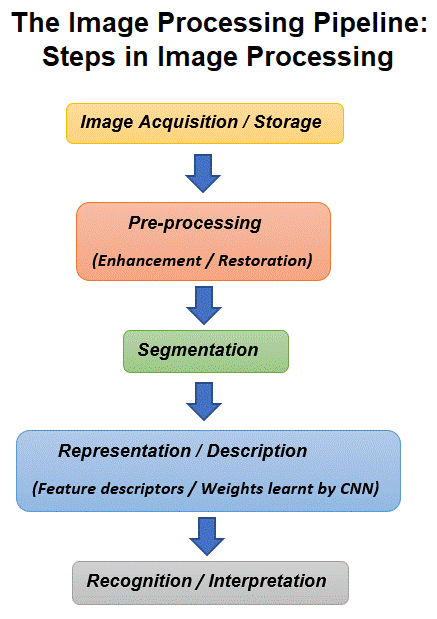The next figure represents different modules that we are going to use for different image processing tasks: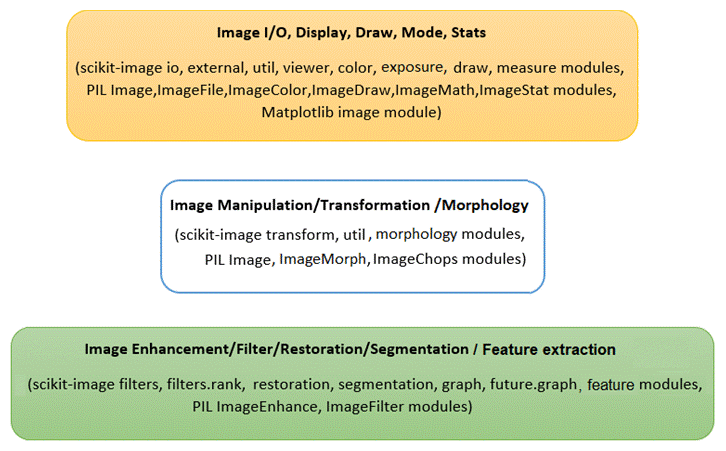Apart from these libraries, we are going to use the following:

• `scipy.ndimage`and`opencv`for different image processing tasks
• `scikit-learn` for classical machine learning
• `tensorflow` and `keras` for deep learning

## Setting up different image processing libraries in Python

The next few paragraphs describe to install different image processing libraries and set up the environment for writing codes to process images using classical image processing techniques in Python. In the last few chapters of this book, we will need to use a different setup when we use deep-learning-based methods.

### Installing pip

We are going to use the `pip`(or`pip3`tool to install the libraries, so—if it isn't already installed—we need to install `pip` first. As mentioned here (https://pip.pypa.io/en/stable/installing/#do-i-need-to-install-pip), `pip` is already installed if we are using Python 3 >=3.4 downloaded from python.org, or if we are working in a  created by `virtualenv` (https://packaging.python.org/key_projects/#virtualenv) or `pyvenv` (https://packaging.python.org/key_projects/#venv). We just need to make sure to upgrade `pip` (https://pip.pypa.io/en/stable/installing/#upgrading-pip). How to install `pip` for different OSes or platforms can be found here: https://stackoverflow.com/questions/6587507/how-to-install-pip-with-python-3.

### Installing some image processing libraries in Python

In Python, there are many libraries that we can use for image processing. The ones we are going to use are: NumPy, SciPy, scikit-image, PIL (Pillow), OpenCV, scikit-learn, SimpleITK, and Matplotlib.

The `matplotlib`library will primarily be used for display purposes, whereas `numpy` will be used for storing an image. The `scikit-learn` library will be used for building machine-learning models for image processing, and `scipy` will be used mainly for image enhancements. The `scikit-image`, `mahotas`, and `opencv` libraries will be used for different image processing algorithms.

The following code block shows how the libraries that we are going to use can be downloaded and installed with `pip` from a Python prompt (interactive mode):

```>>> pip install numpy
>>> pip install scipy
>>> pip install scikit-image
>>> pip install scikit-learn
>>> pip install pillow
>>> pip install SimpleITK
>>> pip install opencv-python
>>> pip install matplotlib```

There may be some additional installation instructions, depending on the OS platform you are going to use. We suggest the reader goes through the documentation sites for each of the libraries to get detailed platform-specific installation instructions for each library. For example, for the `scikit-image` library, detailed installation instructions for different OS platforms can be found here: http://scikit-image.org/docs/stable/install.html. Also, the reader should be familiar with websites such as stackoverflow to resolve platform-dependent installation issues for different libraries.

Finally, we can verify whether a library is properly installed or not by importing it from the Python prompt. If the library is imported successfully (no error message is thrown), then we don't have any installation issue. We can print the version of the library installed by printing it to the console.

The following code block shows the versions for the `scikit-image` and `PIL` Python libraries:

```>>> import skimage, PIL, numpy
>>> print(skimage.__version__)
# 0.14.0
>>> PIL.__version__
# 5.1.0
>>> numpy.__version__
# 1.14.5```

Let us ensure that we have the latest versions of all of the libraries.

### Installing the Anaconda distribution

We also recommend to download and install the latest version of the Anaconda distribution; this will eliminate the need for explicit installation of many Python packages.

### Note

More about installing Anaconda for different OSes can be found at https://conda.io/docs/user-guide/install/index.html.

### Installing Jupyter Notebook

We are going to use Jupyter notebooks to write our Python code. So, we need to install the`jupyter` package first from a Python prompt with `>>> pip install jupyter`, and then launch the Jupyter Notebook app in the browser using `>>> jupyter notebook`. From there, we can create new Python notebooks and choose a kernel. If we use Anaconda, we do not need to install Jupyter explicitly; the latest Anaconda distribution comes with Jupyter.

### Note

We can even install a Python package from inside a notebook cell; for example, we can install`scipy` with the `!pip install scipy` command.

## Image I/O and display with Python

Images are stored as files on the disk, so reading and writing images from the files are disk I/O operations. These can be done using many ways using different libraries; some of them are shown in this section. Let us first start by importing all of the required packages:

```# for inline image display inside notebook
# % matplotlib inline
import numpy as np
from PIL import Image, ImageFont, ImageDraw
from PIL.ImageChops import add, subtract, multiply, difference, screen
import PIL.ImageStat as stat
from skimage import color, viewer, exposure, img_as_float, data
from skimage.transform import SimilarityTransform, warp, swirl
from skimage.util import invert, random_noise, montage
import matplotlib.image as mpimg
import matplotlib.pylab as plt
from scipy.ndimage import affine_transform, zoom
from scipy import misc
```

### Reading, saving, and displaying an image using PIL

The PIL function, `open()`, reads an image from disk in an `Image` object, as shown in the following code. The image is loaded as an object of the `PIL.PngImagePlugin.PngImageFile`class, and we can use properties such as the width, height, and mode to find the size (width x height in pixels or the resolution of the image) and mode of the image:

```im = Image.open("../images/parrot.png") # read the image, provide the correct path
print(im.width, im.height, im.mode, im.format, type(im))
# 453 340 RGB PNG <class 'PIL.PngImagePlugin.PngImageFile'>
im.show() # display the image ```

The following is the output of the previous code: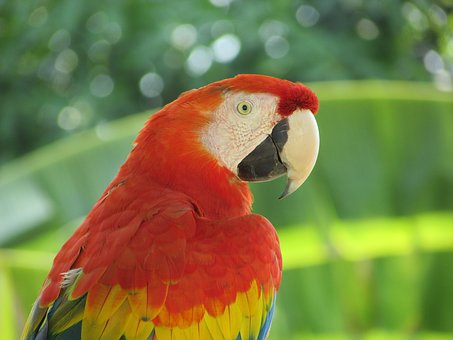The following code block shows how to use the PIL function, `convert()`, to convert the colored RGB image into a grayscale image:

```im_g = im.convert('L')                         # convert the RGB color image to a grayscale image
im_g.save('../images/parrot_gray.png')         # save the image to disk
Image.open("../images/parrot_gray.png").show() # read the grayscale image from disk and show```

The following is the output grayscale image: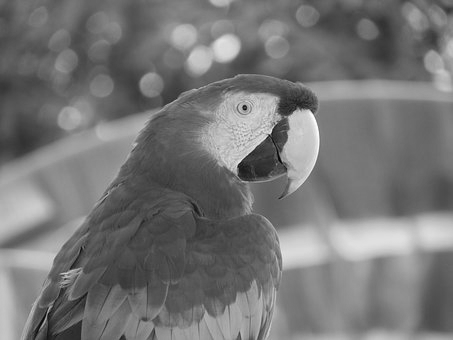#### Providing the correct path to the images on the disk

We recommend creating a folder (sub-directory) to store images to be used for processing (for example, for the Python code samples, we have used the images stored inside a folder named `images`) and then provide the path to the folder to access the image to avoid the `file not found` exception.

### Reading, saving, and displaying an image using Matplotlib

The next code block shows how to use the `imread()`function from`matplotlib.image` to read an image in a floating-point `numpy ndarray`The pixel values are represented as real values between 0 and 1:

```im = mpimg.imread("../images/hill.png")  # read the image from disk as a numpy ndarray
print(im.shape, im.dtype, type(im))      # this image contains an α channel, hence num_channels= 4
# (960, 1280, 4) float32 <class 'numpy.ndarray'>
plt.figure(figsize=(10,10))
plt.imshow(im) # display the image
plt.axis('off')
plt.show()```

The following figure shows the output of the previous code: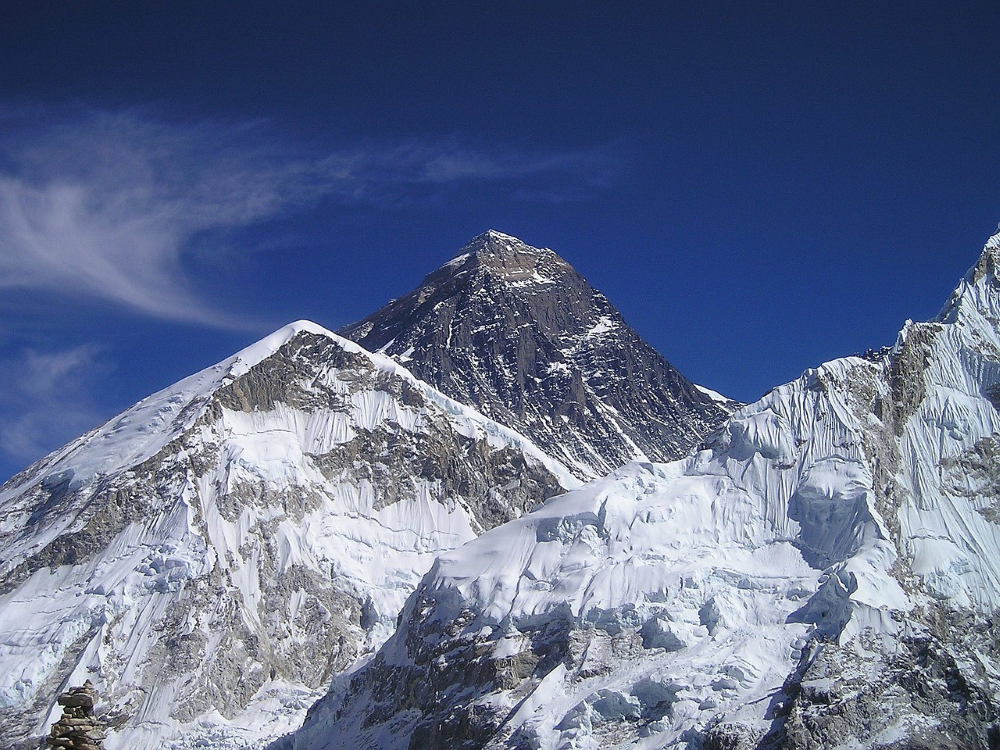The next code snippet changes the image to a darker image by first setting all of the pixel values below 0.5 to 0 and then saving the `numpy ndarray` to disk. The saved image is again reloaded and displayed:

```im1 = im
im1[im1 < 0.5] = 0    # make the image look darker
plt.imshow(im1)
plt.axis('off')
plt.tight_layout()
plt.savefig("../images/hill_dark.png")       # save the dark image
plt.figure(figsize=(10,10))
plt.imshow(im)
plt.axis('off') # no axis ticks
plt.tight_layout()
plt.show()```

The next figure shows the darker image saved with the preceding code: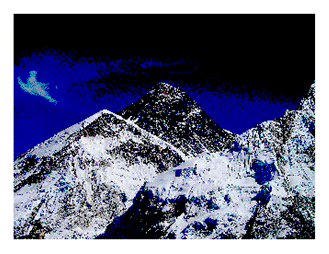#### Interpolating while displaying with Matplotlib imshow()

The `imshow()` function from Matplotlib provides many different types of interpolation methods to plot an image. These functions can be particularly useful when the image to be plotted is small. Let us use the small 50 x 50 `lena` image shown in the next figure to see the effects of plotting with different interpolation methods: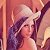The next code block demonstrates how to use different interpolation methods with `imshow()`:

```im = mpimg.imread("../images/lena_small.jpg") # read the image from disk as a numpy ndarray
methods = ['none', 'nearest', 'bilinear', 'bicubic', 'spline16', 'lanczos']
fig, axes = plt.subplots(nrows=2, ncols=3, figsize=(15, 30),
subplot_kw={'xticks': [], 'yticks': []})
for ax, interp_method in zip(axes.flat, methods):
ax.imshow(im, interpolation=interp_method)
ax.set_title(str(interp_method), size=20)
plt.tight_layout()
plt.show()```

The next figure shows the output of the preceding code: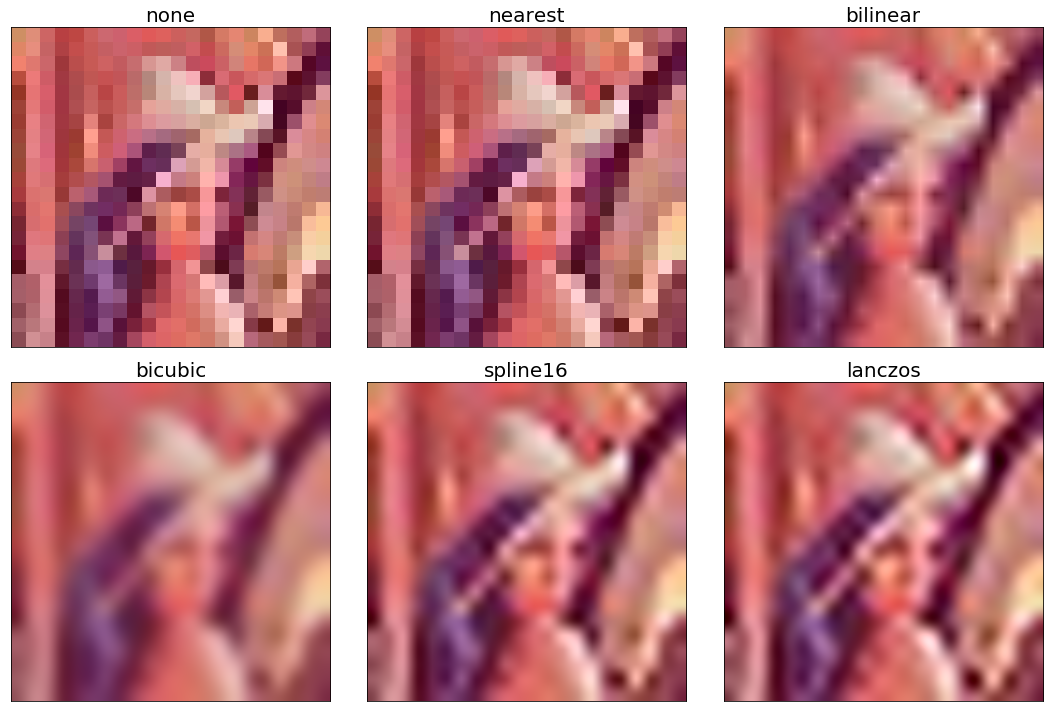### Reading, saving, and displaying an image using scikit-image

The next code block uses the `imread()`function from`scikit-image` to read an image in a `numpy ndarray` of type `uint8` (8-bit unsigned integer). Hence, the pixel values will be in between 0 and 255. Then it converts (changes the image type or mode, which will be discussed shortly) the colored RGB image into an HSV image using the `hsv2rgb()`function from the `Image.color` module. Next, it changes the saturation (colorfulness) to a constant value for all of the pixels by keeping the hue and value channels unchanged. The image is then converted back into RGB mode with the `rgb2hsv()` function to create a new image, which is then saved and displayed:

```im = imread("../images/parrot.png")     # read image from disk, provide the correct path
print(im.shape, im.dtype, type(im))
# (362, 486, 3) uint8 <class 'numpy.ndarray'>
hsv = color.rgb2hsv(im) # from RGB to HSV color space
hsv[:, :, 1] = 0.5 # change the saturation
im1 = color.hsv2rgb(hsv) # from HSV back to RGB
imsave('../images/parrot_hsv.png', im1) # save image to disk
plt.axis('off'), imshow(im), show()```

The next figure shows the output of the previous code—a new image with changed saturation:We can use the scikit-image `viewer` module also to display an image in a pop-up window, as shown in the following code:

```viewer = viewer.ImageViewer(im)
viewer.show()```

#### Using scikit-image's astronaut dataset

The following code block shows how we can load the `astronaut` image from the `scikit-image` library's image datasets with the `data` module. The module contains a few other popular datasets, such as cameraman, which can be loaded similarly:

```im = data.astronaut()
imshow(im), show()```

The next figure shows the output of the preceding code: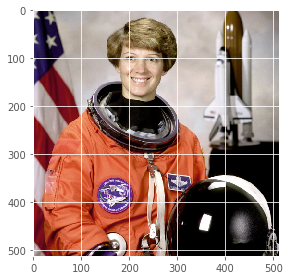#### Reading and displaying multiple images at once

We can use the `imread_collection()` function of the scikit-image `io` module to load in a folder all images that have a particular pattern in the filename and display them simultaneously with the `imshow_collection()` function. The code is left as an exercise for the reader.

### Reading, saving, and displaying an image using scipy misc

The `misc` module of `scipy` can also be used for image I/O and display. The following sections demonstrate how to use the `misc` module functions.

#### Using scipy.misc's face dataset

The next code block shows how to display the `face` dataset of the `misc` module:

```im = misc.face() # load the raccoon's face image
misc.imsave('face.png', im) # uses the Image module (PIL)
plt.imshow(im), plt.axis('off'), plt.show()```

The next figure shows the output of the previous code, which displays the `misc` module's `face` image: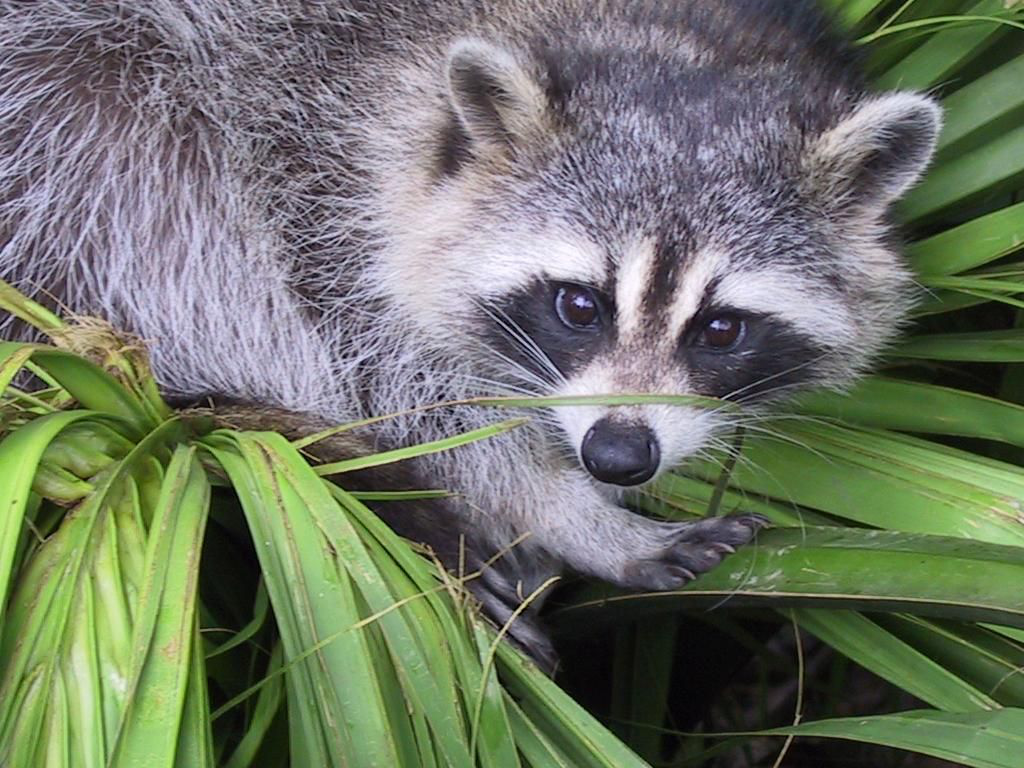We can read an image from disk using `misc.imread()`. The next code block shows an example:

```im = misc.imread('../images/pepper.jpg')
print(type(im), im.shape, im.dtype)
# <class 'numpy.ndarray'> (225, 225, 3) uint8```

The I/O function's `imread()` is deprecated in SciPy 1.0.0, and will be removed in 1.2.0, so the documentation recommends we use the `imageio` library instead. The next code block shows how an image can be read with the `imageio.imread()` function and can be displayed with Matplotlib:

```import imageio
print(type(im), im.shape, im.dtype)
# <class 'imageio.core.util.Image'> (225, 225, 3) uint8
plt.imshow(im), plt.axis('off'), plt.show()```

The next figure shows the output of the previous code block: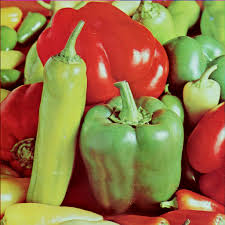## Dealing with different image types and file formats and performing basic image manipulations

In this section, we will discuss different image manipulation functions (with point transformation and geometric transformation) and how to deal with images of different types. Let us start with that.

### Dealing with different image types and file formats

An image can be saved in different file formats and in different modes (types). Let us discuss how to handle images of different file formats and types with Python libraries.

#### File formats

Image files can be of different formats. Some of the popular ones include BMP (8-bit, 24-bit, 32-bit), PNG, JPG (JPEG), GIF, PPM, PNM, and TIFF. We do not need to be worried about the specific format of an image file (and how the metadata is stored) to extract data from it. Python image processing libraries will read the image and extract the data, along with some other useful information for us (for example, image size, type/mode, and data type).

##### Converting from one file format to another

Using PIL, we can read an image in one file format and save it to another; for example, from PNG to JPG, as shown in the following:

```im = Image.open("../images/parrot.png")
print(im.mode)```

```#  RGB```
```im.save("../images/parrot.jpg")```

But if the PNG file is in the `RGBA` mode, we need to convert it into the `RGB` mode before we save it as JPG, as otherwise it will give an error. The next code block shows how to first convert and then save:

```im = Image.open("../images/hill.png")
print(im.mode)
# RGBA
im.convert('RGB').save("../images/hill.jpg") # first convert to RGB mode```

#### Image types (modes)

An image can be of the following different types:

• Single channel images—each pixel is represented by a single value:
• Binary (monochrome) images (each pixel is represented by a single 0-1 bit)
• Gray-level images (each pixel can be represented with 8-bits and can have values typically in the range of 0-255)
• Multi-channel images—each pixel is represented by a tuple of values:
• 3-channel images; for example, the following:
•  RGB images—each pixel is represented by three-tuple (r, g, b) values, representing red, green, and blue channel color values for every pixel.
• HSV images—each pixel is represented by three-tuple (h, s, v) values, representing hue (color), saturation (colorfulness—how much the color is mixed with white), and value (brightness—how much the color is mixed with black) channel color values for every pixel. The HSV model describes colors in a similar manner to how the human eye tends to perceive colors.
• Four-channel images; for example, RGBA images—each pixel is represented by three-tuple (r, g, b, α) values, the last channel representing the transparency.
##### Converting from one image mode into another

We can convert an RGB image into a grayscale image while reading the image itself. The following code does exactly that:

```im = imread("images/parrot.png", as_gray=True)
print(im.shape)
#(362L, 486L)```

Note that we can lose some information while converting into grayscale for some colored images. The following code shows such an example with Ishihara plates, used to detect color-blindness. This time, the `rgb2gray()`function is used from the `color` module, and both the color and the grayscale images are shown side by side. As can be seen in the following figure, the number 8 is almost invisible in the grayscale version:

```im = imread("../images/Ishihara.png")
im_g = color.rgb2gray(im)
plt.subplot(121), plt.imshow(im, cmap='gray'), plt.axis('off')
plt.subplot(122), plt.imshow(im_g, cmap='gray'), plt.axis('off')
plt.show()```

The next figure shows the output of the previous code—the colored image and the grayscale image obtained from it: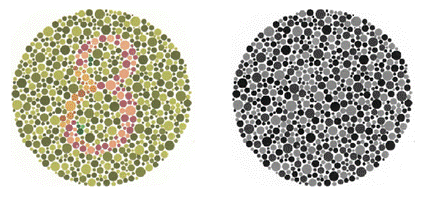#### Some color spaces (channels)

The following represents a few popular channels/color spaces for an image: RGB, HSV, XYZ, YUV, YIQ, YPbPr, YCbCr, and YDbDr. We can use Affine mappings to go from one color space to another. The following matrix represents the linear mapping from the RGB to YIQ color space: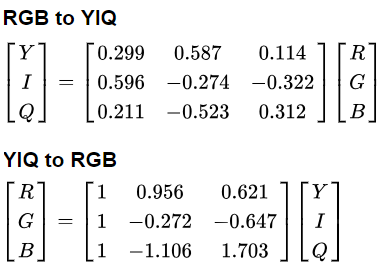##### Converting from one color space into another

We can convert from one color space into another using library functions; for example, the following code converts an RGB color space into an HSV color space image:

```im = imread("../images/parrot.png")
im_hsv = color.rgb2hsv(im)
plt.gray()
plt.figure(figsize=(10,8))
plt.subplot(221), plt.imshow(im_hsv[...,0]), plt.title('h', size=20), plt.axis('off')
plt.subplot(222), plt.imshow(im_hsv[...,1]), plt.title('s', size=20), plt.axis('off')
plt.subplot(223), plt.imshow(im_hsv[...,2]), plt.title('v', size=20), plt.axis('off')
plt.subplot(224), plt.axis('off')
plt.show()```

The next figure shows the h (heu or color: dominant wave length of reflected light), s (saturation or chroma) and v (value or brightness/luminescence) channels of the parrot HSV image, created using the previous code: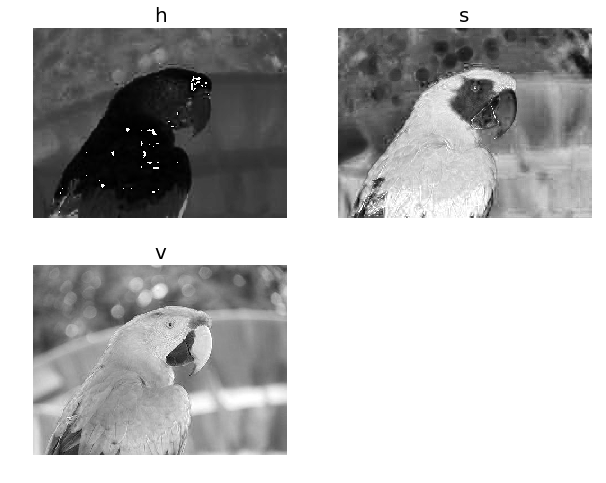Similarly, we can convert the image into the YUV color space using the `rgb2yuv()` function.

#### Data structures to store images

As we have already discussed, PIL uses the `Image` object to store an image, whereas scikit-image uses the `numpy ndarray` data structure to store the image data. The next section describes how to convert between these two data structures.

##### Converting image data structures

The following code block shows how to convert from the PIL `Image` object into `numpy ndarray` (to be consumed by scikit-image):

```im = Image.open('../images/flowers.png') # read image into an Image object with PIL
im = np.array(im) # create a numpy ndarray from the Image object
imshow(im) # use skimage imshow to display the image
plt.axis('off'), show()```

The next figure shows the output of the previous code, which is an image of flowers: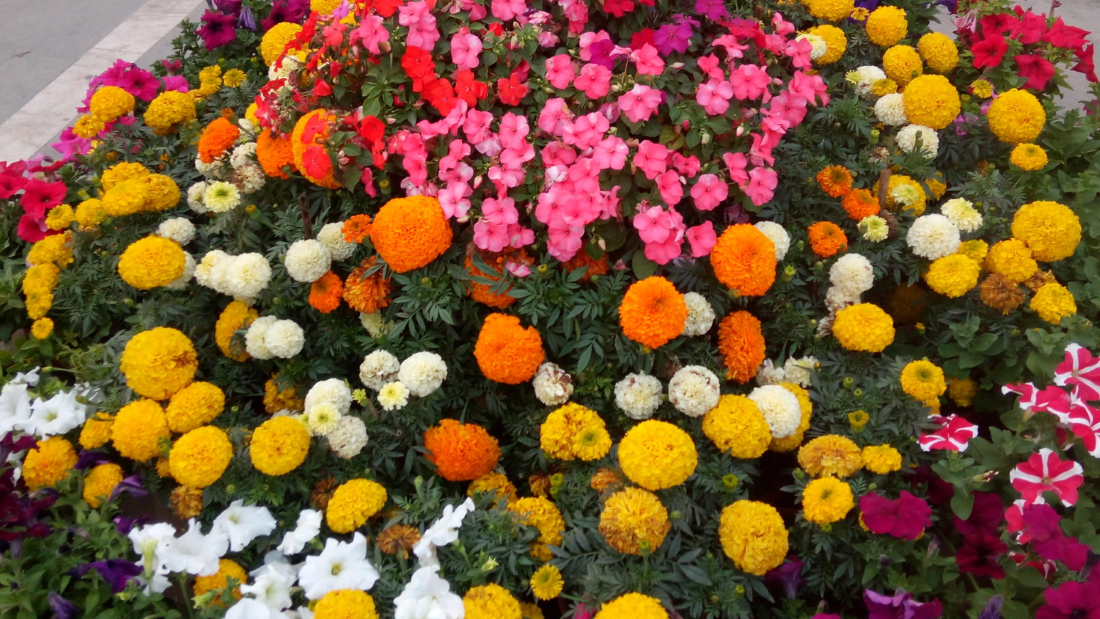The following code block shows how to convert from `numpy ndarray` into a `PIL Image` object. When run, the code shows the same output as the previous figure:

```im = imread('../images/flowers.png') # read image into numpy ndarray with skimage
im = Image.fromarray(im) # create a PIL Image object from the numpy ndarray
im.show() # display the image with PIL Image.show() method```

### Basic image manipulations

Different Python libraries can be used for basic image manipulation. Almost all of the libraries store an image in `numpy ndarray` (a 2-D array for grayscale and a 3-D array for an RGB image, for example). The following figure shows the positive x and y directions (the origin being the top-left corner of the image 2-D array) for the colored `lena` image: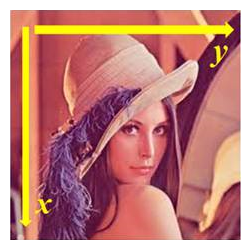#### Image manipulations with numpy array slicing

The next code block shows how slicing and masking with `numpy` arrays can be used to create a circular mask on the `lena` image:

```lena = mpimg.imread("../images/lena.jpg") # read the image from disk as a numpy ndarray
print(lena[0, 40])
# [180  76  83]
# print(lena[10:13, 20:23,0:1]) # slicing
lx, ly, _ = lena.shape
X, Y = np.ogrid[0:lx, 0:ly]
mask = (X - lx / 2) ** 2 + (Y - ly / 2) ** 2 > lx * ly / 4
plt.figure(figsize=(10,10))
plt.imshow(lena), plt.axis('off'), plt.show()```

The following figure shows the output of the code:##### Simple image morphing - α-blending of two images using cross-dissolving

The following code block shows how to start from one face image (image1 being the face of Messi) and end up with another image (image2 being the face of Ronaldo) by using a linear combination of the two image `numpy ndarrays` given with the following equation: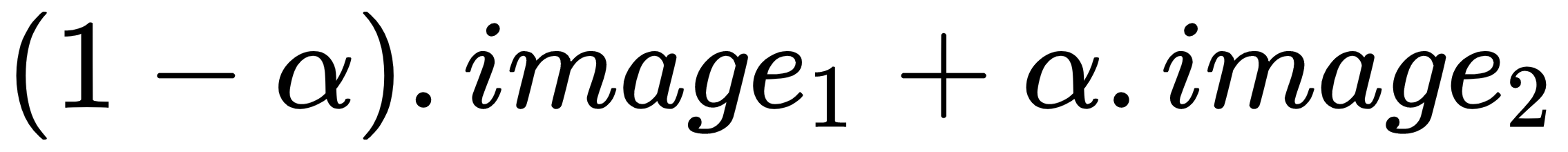We do this by iteratively increasing α from 0 to 1:

```im1 = mpimg.imread("../images/messi.jpg") / 255 # scale RGB values in [0,1]
i = 1
plt.figure(figsize=(18,15))
for alpha in np.linspace(0,1,20):
plt.subplot(4,5,i)
plt.imshow((1-alpha)*im1 + alpha*im2)
plt.axis('off')
i += 1
plt.show()```

The next figure shows the sequence of the α-blended images created using the previous code by cross-dissolving Messi's face image into Ronaldo's. As can be seen from the sequence of intermediate images in the figure, the face morphing with simple blending is not very smooth. In upcoming chapters, we shall see more advanced techniques for image morphing: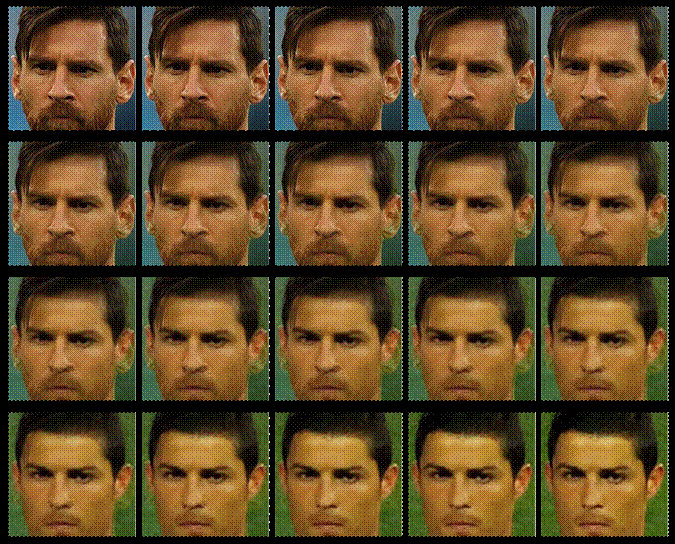#### Image manipulations with PIL

PIL provides us with many functions to manipulate an image; for example, using a point transformation to change pixel values or to perform geometric transformations on an image. Let us first start by loading the parrot PNG image, as shown in the following code:

```im = Image.open("../images/parrot.png")        # open the image, provide the correct path
print(im.width, im.height, im.mode, im.format) # print image size, mode and format
# 486 362 RGB PNG```

The next few sections describe how to do different types of image manipulations with PIL.

##### Cropping an image

We can use the `crop()` function with the desired rectangle argument to crop the corresponding area from the image, as shown in the following code:

```im_c = im.crop((175,75,320,200)) # crop the rectangle given by (left, top, right, bottom) from the image
im_c.show()```

The next figure shows the cropped image created using the previous code: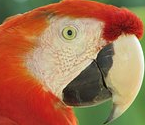##### Resizing an image

In order to increase or decrease the size of an image, we can use the `resize()` function, which internally up-samples or down-samples the image, respectively. This will be discussed in detail in the next chapter.

Resizing to a larger image

Let us start with a small clock image of a size of 149 x 97 and create a larger size image. The following code snippet shows the small clock image we will start with:

```im = Image.open("../images/clock.jpg")
print(im.width, im.height)
# 107 105
im.show()```

The output of the previous code, the small clock image, is shown as follows: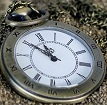The next line of code shows how the `resize()` function can be used to enlarge the previous input clock image (by a factor of 5) to obtain an output image of a size 25 times larger than the input image by using bi-linear interpolation (an up-sampling technique). The details about how this technique works will be described in the next chapter:

`im_large = im.resize((im.width*5, im.height*5), Image.BILINEAR) # bi-linear interpolation`

Resizing to a smaller image

Now let us do the reverse: start with a large image of the Victoria Memorial Hall (of a size of 720 x 540) and create a smaller-sized image. The next code snippet shows the large image to start with:

```im = Image.open("../images/victoria_memorial.png")
print(im.width, im.height)
# 720 540
im.show()```

The output of the previous code, the large image of the Victoria Memorial Hall, is shown as follows: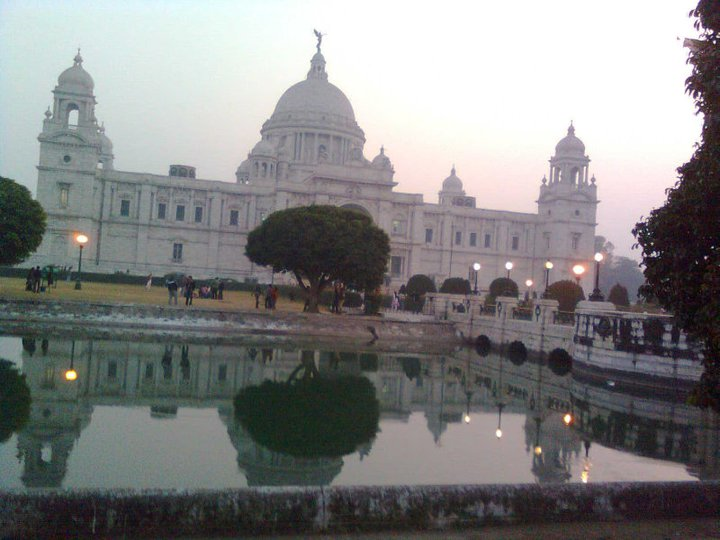The next line of code shows how the `resize()` function can be used to shrink the previous image of the Victoria Memorial Hall (by a factor of 5) to resize it to an output image of a size 25 times smaller than the input image by using anti-aliasing (a high-quality down-sampling technique). We will see how it works in the next chapter:

`im_small = im.resize((im.width//5, im.height//5), Image.ANTIALIAS)`
##### Negating an image

We can use the `point()` function to transform each pixel value with a single-argument function. We can use it to negate an image, as shown in the next code block. The pixel values are represented using 1-byte unsigned integers, which is why subtracting it from the maximum possible value will be the exact point operation required on each pixel to get the inverted image:

```im = Image.open("../images/parrot.png")
im_t = im.point(lambda x: 255 - x)
im_t.show()```

The next figure shows the negative image, the output of the previous code: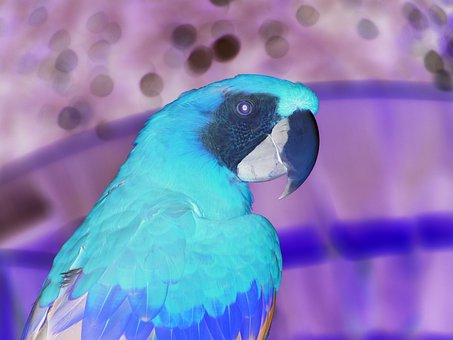##### Converting an image into grayscale

We can use the `convert()` function with the `'L'` parameter to change an RGB color image into a gray-level image, as shown in the following code:

`im_g = im.convert('L')   # convert the RGB color image to a grayscale image`

We are going to use this image for the next few gray-level transformations.

##### Some gray-level transformations

Here we explore a couple of transformations where, using a function, each single pixel value from the input image is transferred to a corresponding pixel value for the output image. The function `point()` can be used for this. Each pixel has a value in between 0 and 255, inclusive.

Log transformation

The log transformation can be used to effectively compress an image that has a dynamic range of pixel values. The following code uses the point transformation for logarithmic transformation. As can be seen, the range of pixel values is narrowed, the brighter pixels from the input image have become darker, and the darker pixels have become brighter, thereby shrinking the range of values of the pixels:

`im_g.point(lambda x: 255*np.log(1+x/255)).show()`

The next figure shows the output log-transformed image produced by running the previous line of code: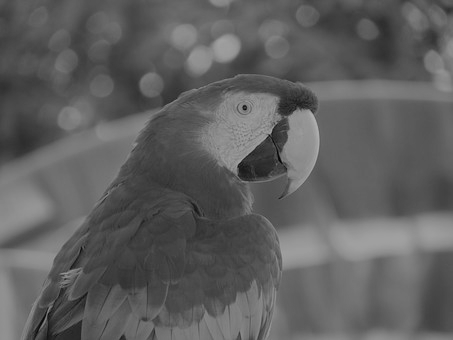Power-law transformation

This transformation is used as γ correction for an image. The next line of code shows how to use the `point()` function for a power-law transformation, where γ = `0.6`:

`im_g.point(lambda x: 255*(x/255)**0.6).show()`

The next figure shows the output power-law-transformed image produced by running the preceding line of code: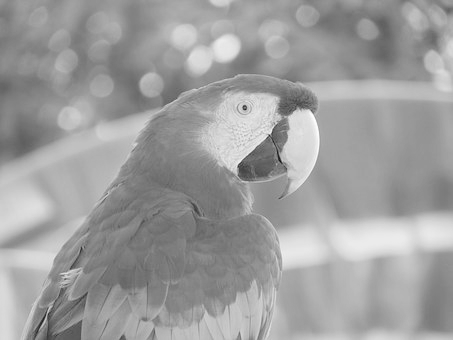##### Some geometric transformations

In this section, we will discuss another set of transformations that are done by multiplying appropriate matrices (often expressed in homogeneous coordinates) with the image matrix. These transformations change the geometric orientation of an image, hence the name.

Reflecting an image

We can use the `transpose()` function to reflect an image with regard to the horizontal or vertical axis:

`im.transpose(Image.FLIP_LEFT_RIGHT).show() # reflect about the vertical axis `

The next figure shows the output image produced by running the previous line of code: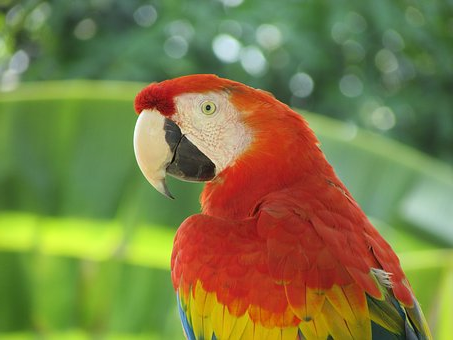Rotating an image

We can use the `rotate()` function to rotate an image by an angle (in degrees):

```im_45 = im.rotate(45) # rotate the image by 45 degrees
im_45.show()          # show the rotated image```

The next figure shows the rotated output image produced by running the preceding line of code: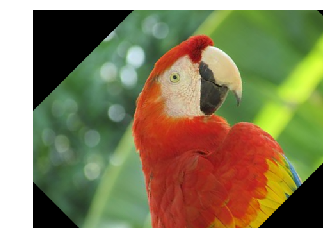Applying an Affine transformation on an image

A 2-D Affine transformation matrix, T, can be applied on each pixel of an image (in homogeneous coordinates) to undergo an Affine transformation, which is often implemented with inverse mapping (warping). An interested reader is advised to refer to this article (https://sandipanweb.wordpress.com/2018/01/21/recursive-graphics-bilinear-interpolation-and-image-transformation-in-python/) to understand how these transformations can be implemented (from scratch).

The following code shows the output image obtained when the input image is transformed with a shear transform matrix. The data argument in the `transform()` function is a 6-tuple (a, b, c, d, e, f), which contains the first two rows from an Affine transform matrix. For each pixel (x, y) in the output image, the new value is taken from a position (a x + b y + c, d x + e y + f) in the input image, which is rounded to nearest pixel. The `transform()` function can be used to scale, translate, rotate, and shear the original image:

```im = Image.open("../images/parrot.png")
im.transform((int(1.4*im.width), im.height), Image.AFFINE, data=(1,-0.5,0,0,1,0)).show() # shear```

The next figure shows the output image with shear transform, produced by running the previous code: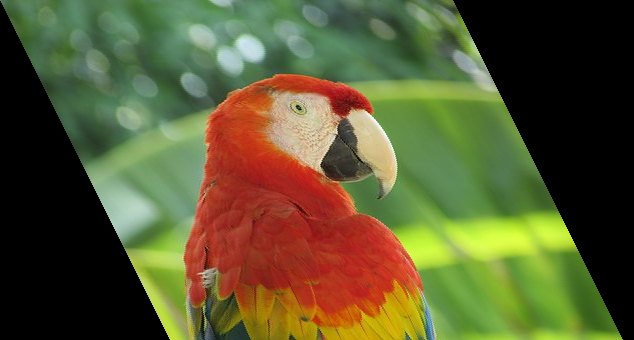Perspective transformation

We can run a perspective transformation on an image with the `transform()` function by using the `Image.PERSPECTIVE` argument, as shown in the next code block:

```params = [1, 0.1, 0, -0.1, 0.5, 0, -0.005, -0.001]
im1 = im.transform((im.width//3, im.height), Image.PERSPECTIVE, params, Image.BICUBIC)
im1.show()```

The next figure shows the image obtained after the perspective projection, by running the preceding code block: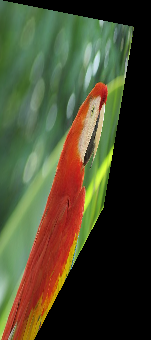##### Changing pixel values of an image

We can use the `putpixel()` function to change a pixel value in an image. Next, let us discuss a popular application of adding noise to an image using the function.

Adding salt and pepper noise to an image

We can add some salt-and-pepper noise to an image by selecting a few pixels from the image randomly and then setting about half of those pixel values to black and the other half to white. The next code snippet shows how to add the noise:

```# choose 5000 random locations inside image
im1 = im.copy() # keep the original image, create a copy
n = 5000
x, y = np.random.randint(0, im.width, n), np.random.randint(0, im.height, n)
for (x,y) in zip(x,y):
im1.putpixel((x, y), ((0,0,0) if np.random.rand() < 0.5 else (255,255,255))) # salt-and-pepper noise
im1.show()```

The following figure shows the output noisy image generated by running the previous code: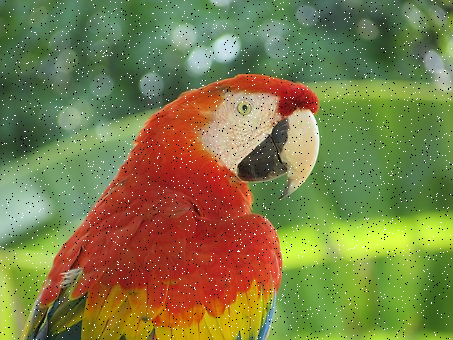##### Drawing on an image

We can draw lines or other geometric shapes on an image (for example, the `ellipse()` function to draw an ellipse) from the `PIL.ImageDraw` module, as shown in the next Python code snippet:

```im = Image.open("../images/parrot.png")
draw = ImageDraw.Draw(im)
draw.ellipse((125, 125, 200, 250), fill=(255,255,255,128))
del draw
im.show()```

The following figure shows the output image generated by running the previous code: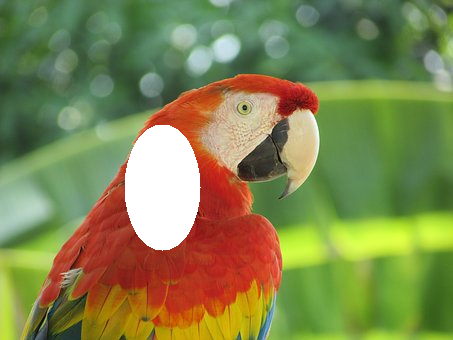##### Drawing text on an image

We can add text to an image using the `text()` function from the `PIL.ImageDraw` module, as shown in the next Python code snippet:

```draw = ImageDraw.Draw(im)
font = ImageFont.truetype("arial.ttf", 23) # use a truetype font
draw.text((10, 5), "Welcome to image processing with python", font=font)
del draw
im.show()```

The following figure shows the output image generated by running the previous code: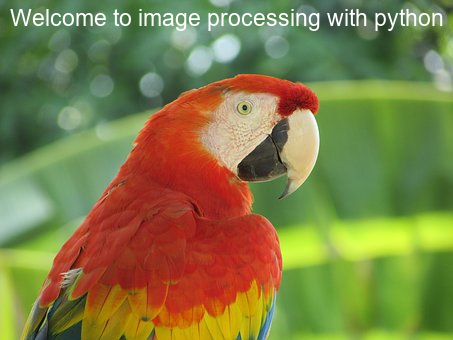##### Creating a thumbnail

We can create a thumbnail from an image with the `thumbnail()` function, as shown in the following:

```im_thumbnail = im.copy() # need to copy the original image first
im_thumbnail.thumbnail((100,100))
# now paste the thumbnail on the image
im.paste(im_thumbnail,(10,10))im.save("../images/parrot_thumb.jpg")im.show()```

The figure shows the output image generated by running the preceding code snippet: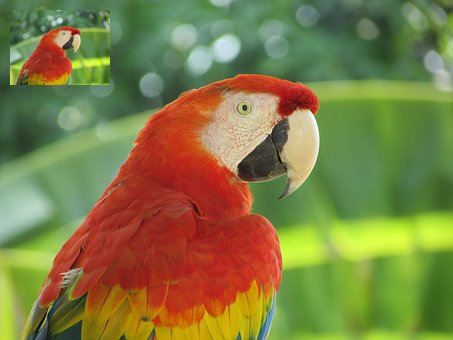##### Computing the basic statistics of an image

We can use the `stat` module to compute the basic statistics (mean, median, standard deviation of pixel values of different channels, and so on) of an image, as shown in the following:

```s = stat.Stat(im)
print(s.extrema) # maximum and minimum pixel values for each channel R, G, B
# [(4, 255), (0, 255), (0, 253)]
print(s.count)
# [154020, 154020, 154020]
print(s.mean)
# [125.41305674587716, 124.43517724970783, 68.38463186599142]
print(s.median)
# [117, 128, 63]
print(s.stddev)
# [47.56564506512579, 51.08397900881395, 39.067418896260094]```
##### Plotting the histograms of pixel values for the RGB channels of an image

The `histogram()` function can be used to compute the histogram (a table of pixel values versus frequencies) of pixels for each channel and return the concatenated output (for example, for an RGB image, the output contains 3 x 256 = 768 values):

```pl = im.histogram()
plt.bar(range(256), pl[:256], color='r', alpha=0.5)
plt.bar(range(256), pl[256:2*256], color='g', alpha=0.4)
plt.bar(range(256), pl[2*256:], color='b', alpha=0.3)
plt.show()```

The following figure shows the R, G, and B color histograms plotted by running the previous code: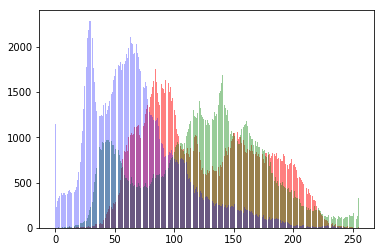##### Separating the RGB channels of an image

We can use the `split()` function to separate the channels of a multi-channel image, as is shown in the following code for an RGB image:

```ch_r, ch_g, ch_b = im.split() # split the RGB image into 3 channels: R, G and B
# we shall use matplotlib to display the channels
plt.figure(figsize=(18,6))
plt.subplot(1,3,1); plt.imshow(ch_r, cmap=plt.cm.Reds); plt.axis('off')
plt.subplot(1,3,2); plt.imshow(ch_g, cmap=plt.cm.Greens); plt.axis('off')
plt.subplot(1,3,3); plt.imshow(ch_b, cmap=plt.cm.Blues); plt.axis('off')
plt.tight_layout()
plt.show() # show the R, G, B channels```

The following figure shows three output images created for each of the R (red), G (green), and B (blue) channels generated by running the previous code: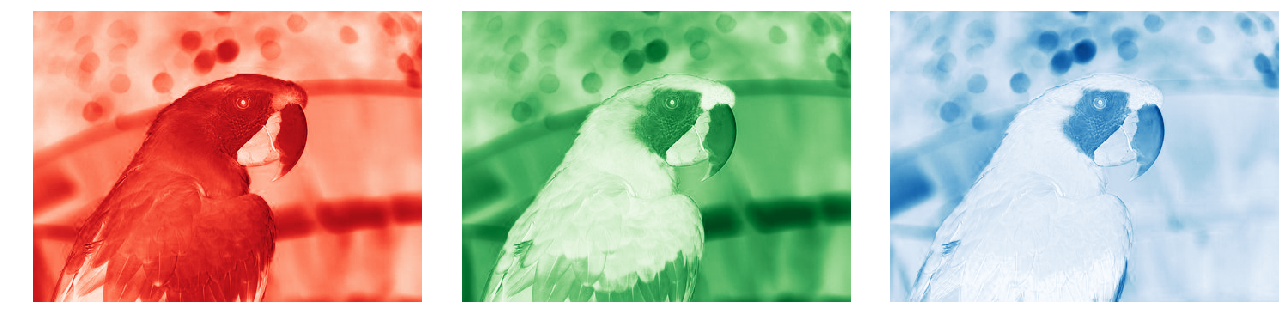##### Combining multiple channels of an image

We can use the`merge()`function to combine the channels of a multi-channel image, as is shown in the following code, wherein the color channels obtained by splitting the parrot RGB image are merged after swapping the red and blue channels:

```im = Image.merge('RGB', (ch_b, ch_g, ch_r)) # swap the red and blue channels obtained last time with split()
im.show()```

The following figure shows the RGB output image created by merging the B, G, and R channels by running the preceding code snippet: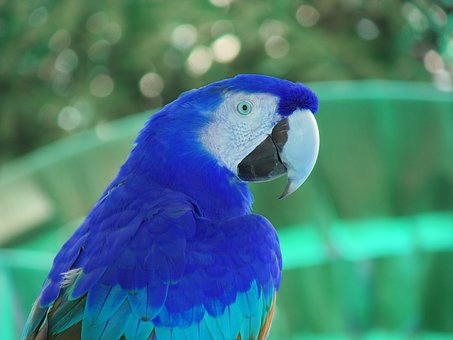##### α-blending two images

The `blend()` function can be used to create a new image by interpolating two given images (of the same size) using a constant, α. Both images must have the same size and mode. The output image is given by the following:

out = image1 * (1.0 - α) + image2 * α

If α is 0.0, a copy of the first image is returned. If α is 1.0, a copy of the second image is returned. The next code snippet shows an example:

```im1 = Image.open("../images/parrot.png")
im2 = Image.open("../images/hill.png")
# 453 340 1280 960 RGB RGBA
im1 = im1.convert('RGBA') # two images have different modes, must be converted to the same mode
im2 = im2.resize((im1.width, im1.height), Image.BILINEAR) # two images have different sizes, must be converted to the same size
im = Image.blend(im1, im2, alpha=0.5).show()```

The following figure shows the output image generated by blending the previous two images: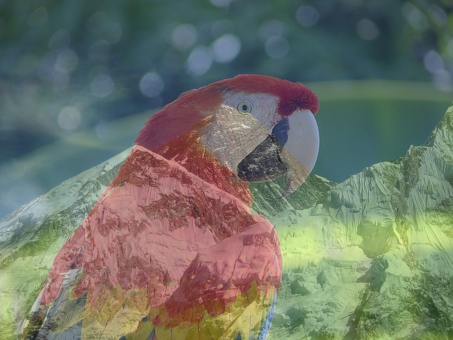##### Superimposing two images

An image can be superimposed on top of another by multiplying two input images (of the same size) pixel by pixel. The next code snippet shows an example:

```im1 = Image.open("../images/parrot.png")
im2 = Image.open("../images/hill.png").convert('RGB').resize((im1.width, im1.height))
multiply(im1, im2).show()```

The next figure shows the output image generated when superimposing two images by running the preceding code snippet: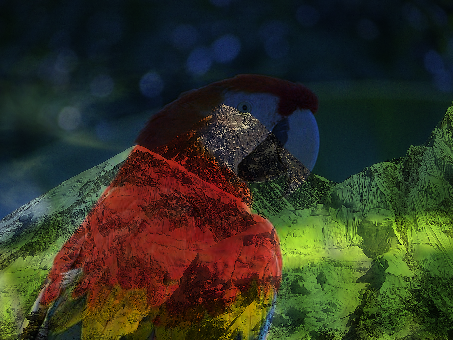The next code snippet shows how an image can be generated by adding two input images (of the same size) pixel by pixel:

`add(im1, im2).show()`

The next figure shows the output image generated by running the previous code snippet: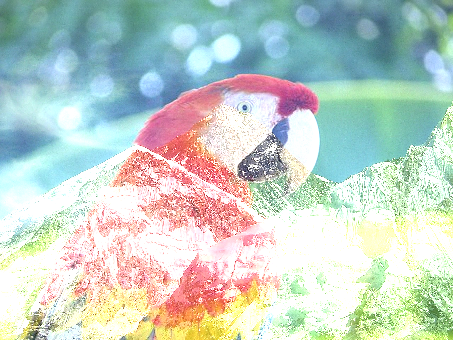##### Computing the difference between two images

The following code returns the absolute value of the pixel-by-pixel difference between images. Image difference can be used to detect changes between two images. For example, the next code block shows how to compute the difference image from two successive frames from a video recording (from YouTube) of a match from the 2018 FIFA World Cup:

```from PIL.ImageChops import subtract, multiply, screen, difference, add
im1 = Image.open("../images/goal1.png") # load two consecutive frame images from the video
im2 = Image.open("../images/goal2.png")
im = difference(im1, im2)
im.save("../images/goal_diff.png")

plt.subplot(311)
plt.imshow(im1)
plt.axis('off')
plt.subplot(312)
plt.imshow(im2)
plt.axis('off')
plt.subplot(313)
plt.imshow(im), plt.axis('off')
plt.show()```

The next figure shows the output of the code, with the consecutive frame images followed by their difference image:

First frame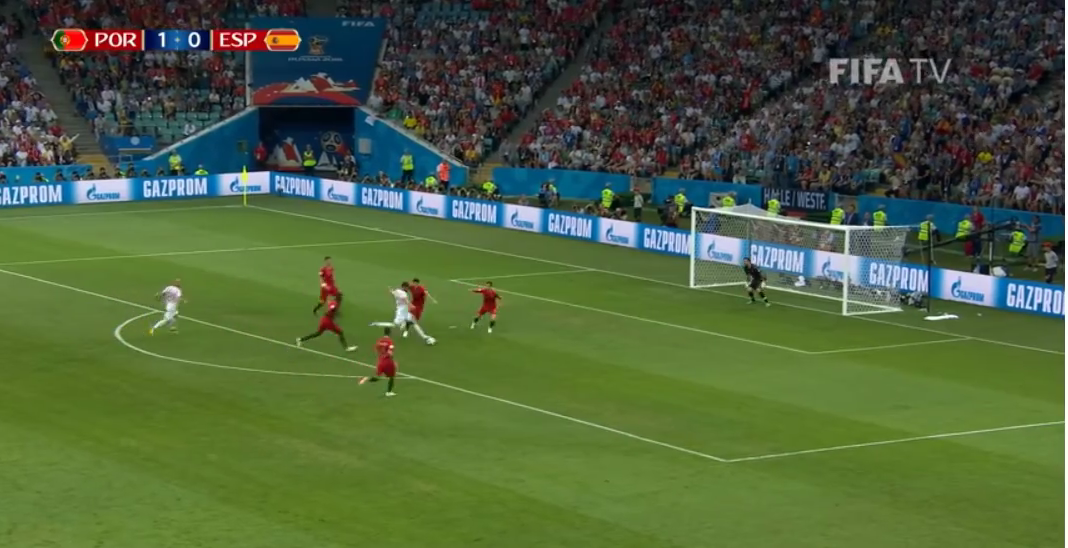Second frame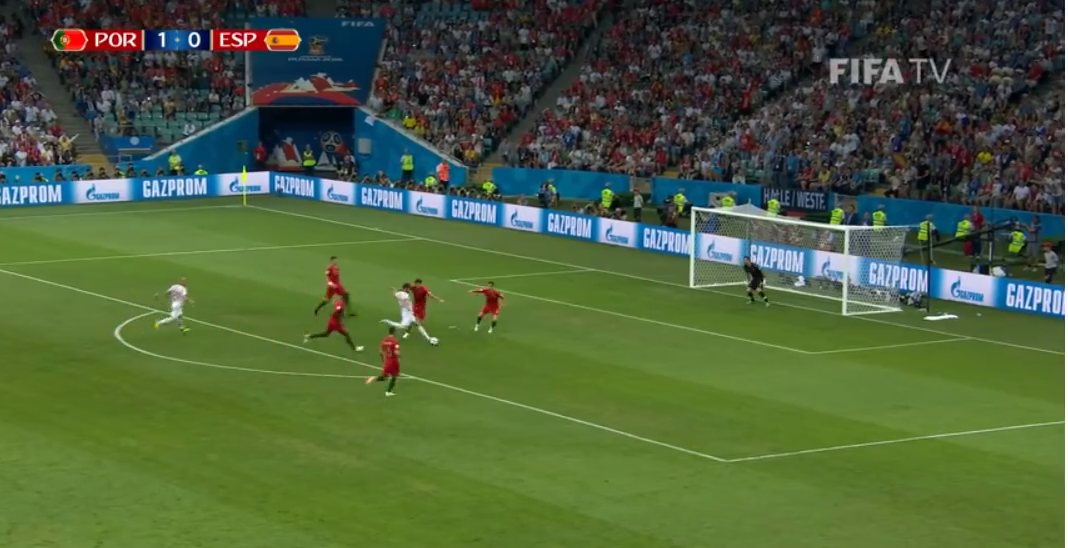The difference image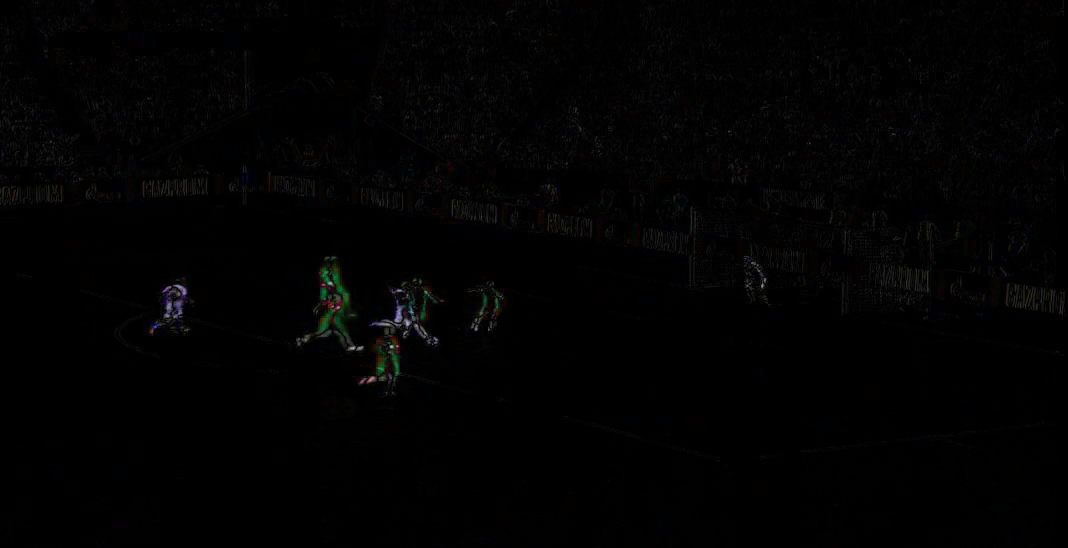##### Subtracting two images and superimposing two image negatives

The `subtract()` function can be used to first subtract two images, followed by dividing the result by scale (defaults to 1.0) and adding the offset (defaults to 0.0). Similarly, the `screen()` function can be used to superimpose two inverted images on top of each other.

#### Image manipulations with scikit-image

As done previously using the PIL library, we can also use the `scikit-image` library functions for image manipulation. Some examples are shown in the following sections.

##### Inverse warping and geometric transformation using the warp() function

The scikit-image `transform` module's `warp()` function can be used for inverse warping for the geometric transformation of an image (discussed in a previous section), as demonstrated in the following examples.

Applying an Affine transformation on an image

We can use the `SimilarityTransform()` function to compute the transformation matrix, followed by `warp()` function, to carry out the transformation, as shown in the next code block:

```im = imread("../images/parrot.png")
tform = SimilarityTransform(scale=0.9, rotation=np.pi/4,translation=(im.shape/2, -100))
warped = warp(im, tform)
import matplotlib.pyplot as plt
plt.imshow(warped), plt.axis('off'), plt.show()```

The following figure shows the output image generated by running the previous code snippet: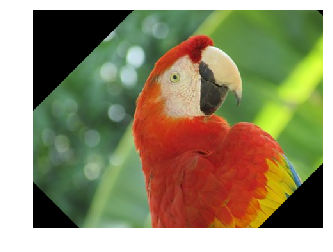##### Applying the swirl transform

This is a non-linear transform defined in the scikit-image documentation. The next code snippet shows how to use the `swirl()`function to implement the transform, where `strength` is a parameter to the function for the amount of `swirl``radius` indicates the `swirl` extent in pixels, and `rotation` adds a rotation angle. The transformation of `radius` into `r` is to ensure that the transformation decays to ≈ 1/1000t≈ 1/1000th within the specified radius:

```im = imread("../images/parrot.png")
swirled = swirl(im, rotation=0, strength=15, radius=200)
plt.imshow(swirled)
plt.axis('off')
plt.show()```

The next figure shows the output image generated with swirl transformation by running the previous code snippet: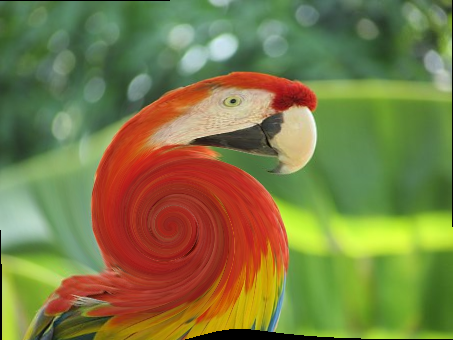##### Adding random Gaussian noise to images

We can use the `random_noise()` function to add different types of noise to an image. The next code example shows how Gaussian noise with different variances can be added to an image:

```im = img_as_float(imread("../images/parrot.png"))
plt.figure(figsize=(15,12))
sigmas = [0.1, 0.25, 0.5, 1]
for i in range(4):
noisy = random_noise(im, var=sigmas[i]**2)
plt.subplot(2,2,i+1)
plt.imshow(noisy)
plt.axis('off')
plt.title('Gaussian noise with sigma=' + str(sigmas[i]), size=20)
plt.tight_layout()
plt.show()```

The next figure shows the output image generated by adding Gaussian noises with different variance by running the previous code snippet. As can be seen, the more the standard deviation of the Gaussian noise, the noisier the output image: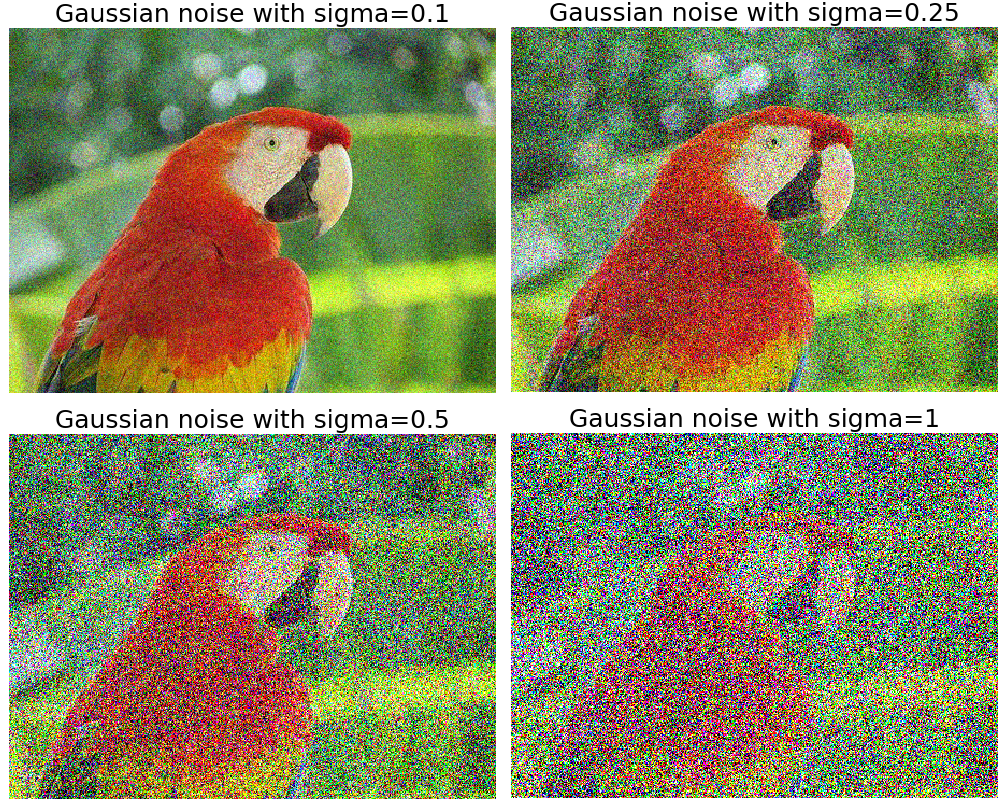##### Computing the cumulative distribution function of an image

We can compute the cumulative distribution function (CDF) for a given image with the `cumulative_distribution()` function, as we shall see in the image enhancement chapter. For now, the reader is encouraged to find the usage of this function to compute the CDF.

#### Image manipulation with Matplotlib

We can use the `pylab` module from the `matplotlib` library for image manipulation. The next section shows an example.

##### Drawing contour lines for an image

A contour line for an image is a curve connecting all of the pixels where they have the same particular value. The following code block shows how to draw the contour lines and filled contours for a grayscale image of Einstein:

```im = rgb2gray(imread("../images/einstein.jpg")) # read the image from disk as a numpy ndarray
plt.figure(figsize=(20,8))
plt.subplot(131), plt.imshow(im, cmap='gray'), plt.title('Original Image', size=20)
plt.subplot(132), plt.contour(np.flipud(im), colors='k', levels=np.logspace(-15, 15, 100))
plt.title('Image Contour Lines', size=20)
plt.subplot(133), plt.title('Image Filled Contour', size=20), plt.contourf(np.flipud(im), cmap='inferno')
plt.show()```

The next figure shows the output of the previous code: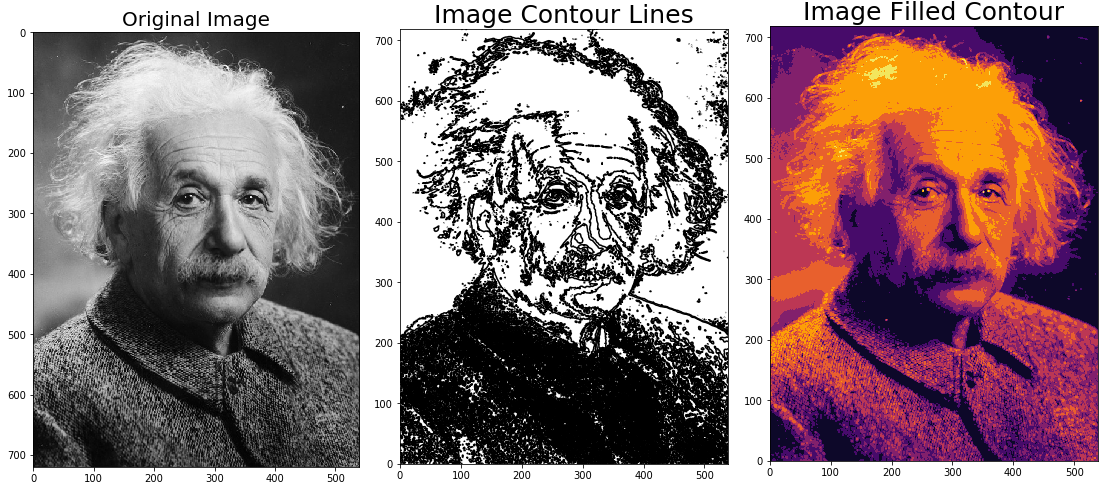#### Image manipulation with the scipy.misc and scipy.ndimage modules

We can use the `misc` and `ndimage` modules from the `scipy` library too for image manipulation; it is left as an exercise for the reader to find the relevant function and get familiar with their usage.

## Summary

In this chapter, we first provided a basic introduction to image processing and basic concepts regarding the problems that we try to solve in image processing. We then discussed different tasks and steps with image processing, and the leading image processing libraries in Python, which we are going to use for coding in this book. Next, we talked about how to install different libraries for image processing in Python, and how to import them and call the functions from the modules. We also covered basic concepts about image types, file formats, and data structures to store image data with different Python libraries. Then, we discussed how to perform image I/O and display in Python using different libraries. Finally, we discussed how to perform basic image manipulations with different Python libraries. In the next chapter, we will deep dive into sampling, quantization, convolution, the Fourier transform, and frequency domain filtering on images.

## Questions

1. Use the `scikit-image` library's functions to read a collection of images and display them as a montage.
2. Use the `scipy ndimage` and `misc` modules' functions to zoom, crop, resize, and apply Affine transformation to an image.
3. Create a Python remake of the Gotham Instagram filter (https://github.com/lukexyz/CV-Instagram-Filters) (hint: manipulate an image with the PIL `split()`, `merge()`, and `numpy interp()` functions to create a channel interpolation (https://www.youtube.com/watch?v=otLGDpBglEA&feature=player_embedded)).
4. Use scikit-image's `warp()` function to implement the swirl transform. Note that the `swirl` transform can also be expressed with the following equations: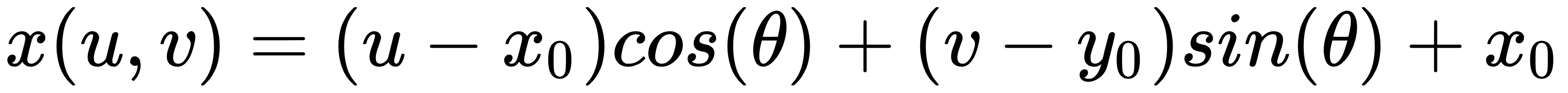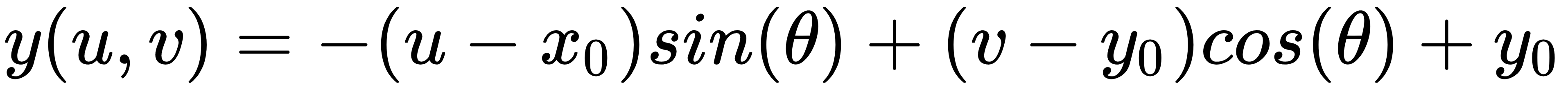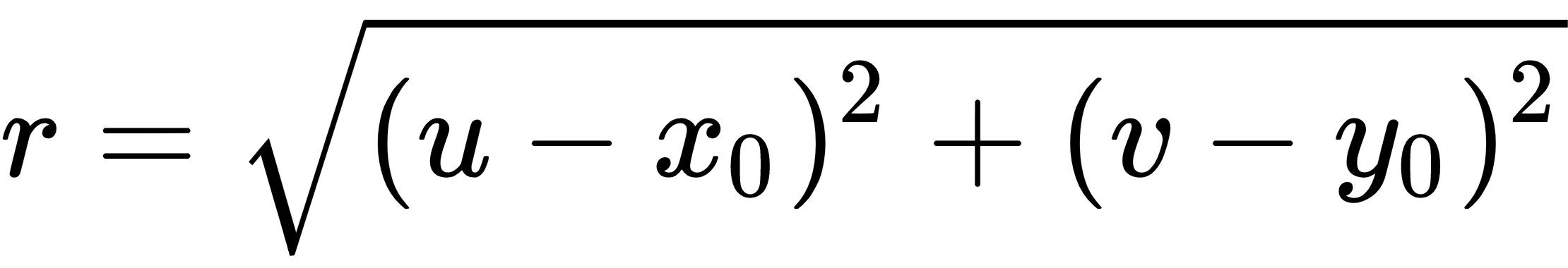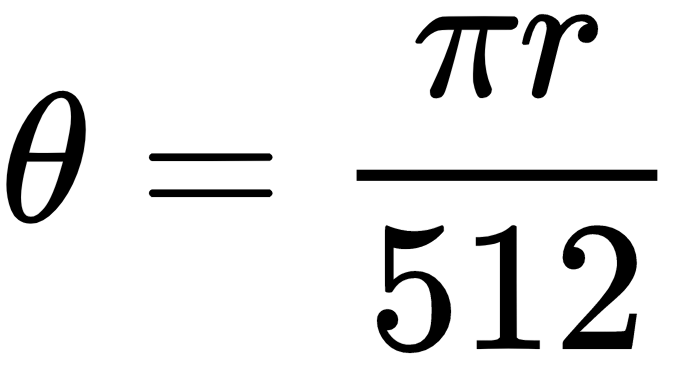1. Implement the wave transform (hint: use scikit-image's `warp()`) given by the following: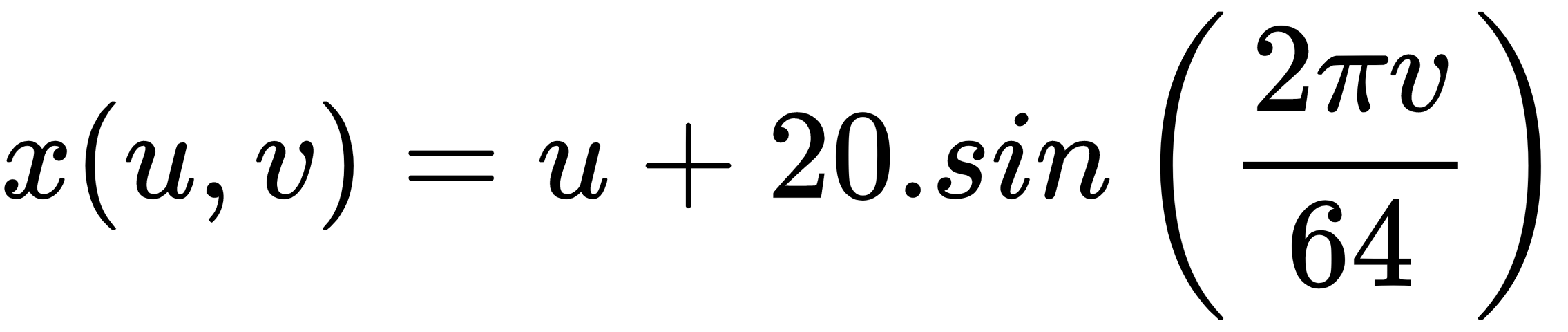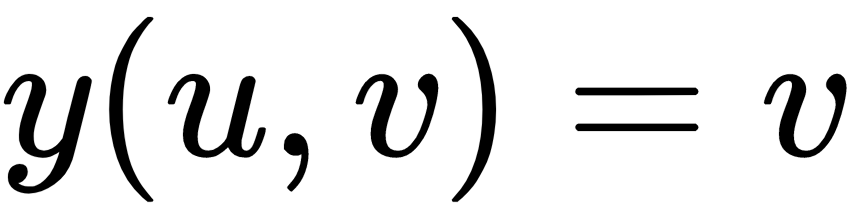1. Use PIL to load an RGB `.png` file with a palette and convert into a grayscale image. This problem is taken from this post: https://stackoverflow.com/questions/51676447/python-use-pil-to-load-png-file-gives-strange-results/51678271#51678271. Convert the following RGB image (from the `VOC2012` dataset) into a grayscale image by indexing the palette: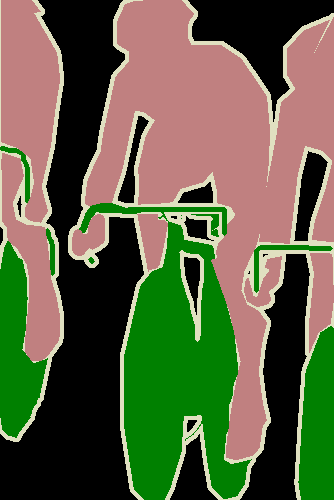1. Make a 3D plot for each of the color channels of the parrot image used in this chapter (hint: use the `mpl_toolkits.mplot3d` module's `plot_surface()` function and NumPy's `meshgrid()` function).
1. Use scikit-image's `transform` module's `ProjectiveTransform` to estimate the homography matrix from a source to a destination image and use the `inverse()` function to embed the Lena image (or yours) in the blank canvas as shown in the following:
 Input Image Output ImageFirst try to solve the problems on your own. For your reference, the solutions can be found here: https://sandipanweb.wordpress.com/2018/07/30/some-image-processing-problems/.